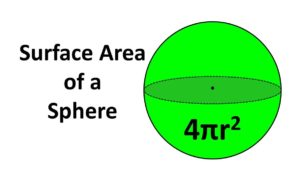# Total Surface Area Of Sphere Java Program | Programs

Java Program for finding Total surface area of a sphere. Here we have discussed about the various methods to calculate the Total Surface Area Of Sphere such as using the standard formula, Command Line Arguments and Class name in the main method. The compiler is added to each program mentioned with sample outputs.

Sphere is a completely round object in a 3-dimensional space.The formula for calculating the Surface Area of a sphere with radius ‘r’ would be 4πr².

## Using Standard Formula

1) We are calculating the total surface area of sphere using the standard formula is area=(4*22*r*r)/(7).

2) Read the radius value using scanner object sc.nextDouble() and store it in the variable r.Substitute the value in the formula and print the area value.

Output:

### Using Command Line Arguments

1) In this program we are reading the radius value using command line arguments.

2) The values we will pass  at run-time are called command line arguments, will store at string array “String args[]” of main method.

3) We are converting the value available at index 0 which is string, in to double using Double.parseDouble(). Here  Double is wrapper class and store that value  in the variable r, and calculate the area and print  the total surface area of sphere

Output:

#### Using Class Name in the Main Method

1)We are calling the static method with its class name in the main method as TotalSurfaceAreaOfSphere.area(r); then static method executed and returns the area value and that value will be assigned to a and print the total surface area of the sphere.

Output:

More Programs:

x

## Plus Star Pattern Java Program | Patterns

Java program to print plus star pattern program – We have written the below print/draw ...